Study Guide

# Electric Fields

## Electric Fields

Every charged particle interacts with other charged particles via its electric field. An electric field is the invisible force field that charges create, the same way earth creates a gravitational field. Electric fields may not be able to stop incoming Klingon photon torpedoes, but they do allow charges to push and pull other charges without touching them. Which is almost as good, right?

The field (E) that a charged particle (q) creates will get less and less strong as you move further and further away from it, given by the formula: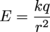You may think this looks really similar to Coulomb's Law—kudos. In fact, we can interpret Coulomb's Law as really telling us the force on one charge that's sitting in the electric field of a second charge:

Fe = qE

This means E is measured in newtons per coulomb. We can increase Fe either by increasing the field or by increasing the charge.

The strength of an electric field is determined by the medium the charge is placed in—a charge in air will create a different magnitude field than a charge in, say, water. This is called the permittivity of the medium, and we'll almost always deal with the permittivity of free space, which is to say vacuum (or, for all intents and purposes, air, which is just 1.0006 times that of vacuum).

The permittivity of free space is given by the electric constant,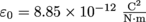.We can rewrite our formula for electric field in terms of the permittivity of the material the charge is placed in, because there is a direct relationship between Coulomb's constant and the electric constant: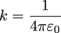This gives us: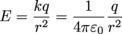### Field Lines and Flux

Fields like the electric field are very easy to represent with simple drawings, so get your sketchpad ready. A charged particle will create a field that radiates outward (positive charge) or inward (negative charge), in every direction: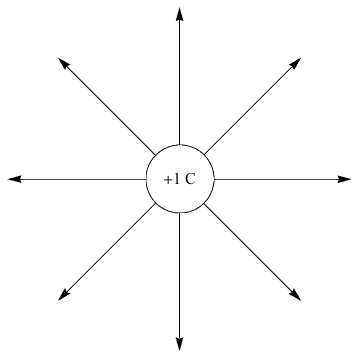The bigger the charge, the more field lines it will create (and the stronger the field):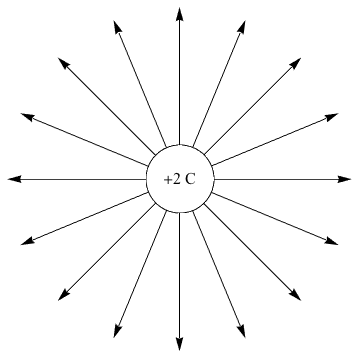You can imagine drawing circles at various values of r away from the charge. The further away you get, the less dense the field lines are—this is exactly what our equation for E tells us. The field line density at any point is called the electric fluxE), a measure of how strong a field is over a given surface area:

ΦE = EA

Remember that here A is surface area, because even if it's tricky to draw, charges radiates field lines in every direction, not just those on your paper. ΦE will be 0 if no field lines go through that area.

The fun part of drawing fields comes when we have multiple charges. Opposite charges' fields will attract, bending the two sets of field lines toward each other: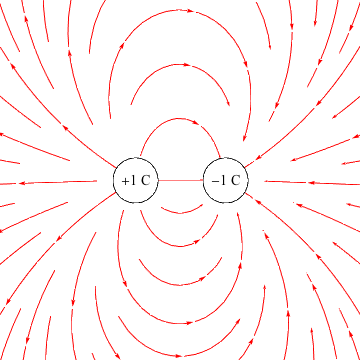While like charges' fields will repel: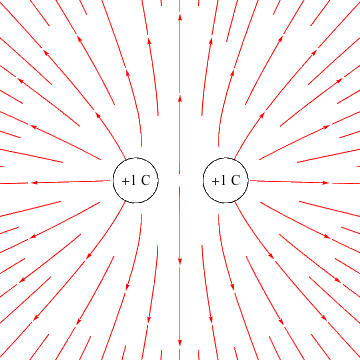Look at all those happy little fields!

### Gauss' Law

A more quantitative way to calculate exactly how happy a field is (sorry, Ross) is Gauss' Law. The amount of electric flux a charge q generates is constant, and depends only on the size of the charge: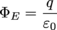This is commonly written in terms of the electric field and surface area around the charge: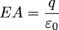What Gauss' Law says is that at any distance away from a charge q, the net flux radiating outwards is the same. The field is definitely not the same—we know it gets weaker as we get further away from the charge—but it falls off as r2, which is exactly the rate at which the surface area the field is measured over increases, keeping the net flux constant.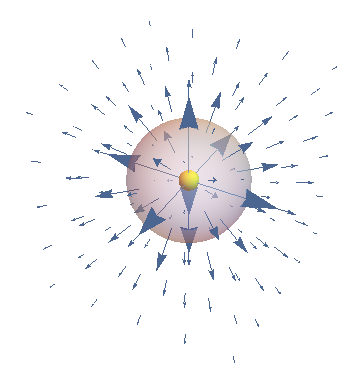Gauss' Law says if we know q, we can find E a distance r away. A Gaussian surface refers to an imaginary box, disk, ball, donut, pot roast or any other shape that entirely encloses the charge that we'll take the surface area of to do calculations. We'll stick to disks or balls as it makes the math easier. We'll just draw a Gaussian surfacearound the charge—in this case, an imaginary sphere with radius r. We have:Which is exactly our formula for E a distance r away from a charge q. Gauss knew a thing or two about physics, after all.

### Common Mistakes

The hardest part of a problem using Gauss' Law is usually figuring out exactly what kind of Gaussian surface to draw. Nine times out of ten, it's either going to be a sphere (point charges, charged spheres, etc.) or a cylinder (charged rods, charged sheets, conductors, etc.).

### Brain Snack

As if it wasn't weird enough already, the platypus is one of a small handful of mammals that can detect changes in electric fields caused by its prey's muscle contractions3. It then uses this sense and its knowledge of Gauss' Law to locate its prey precisely in murky water.